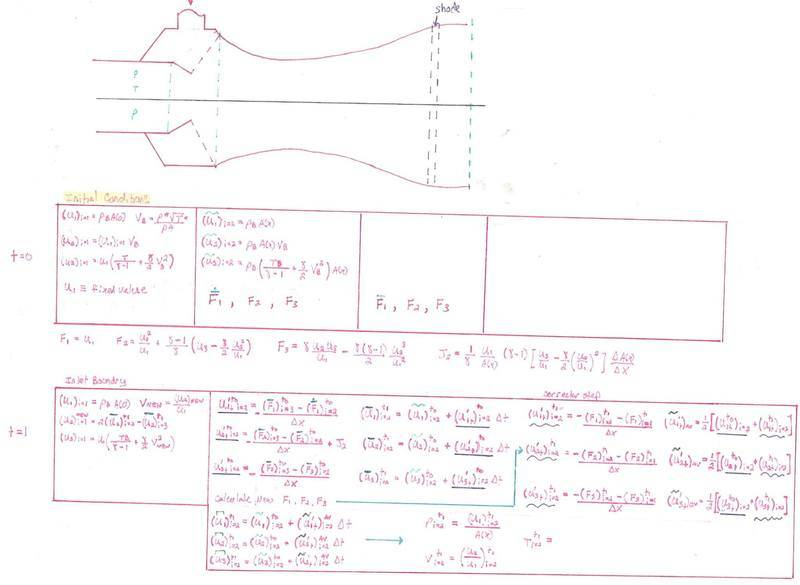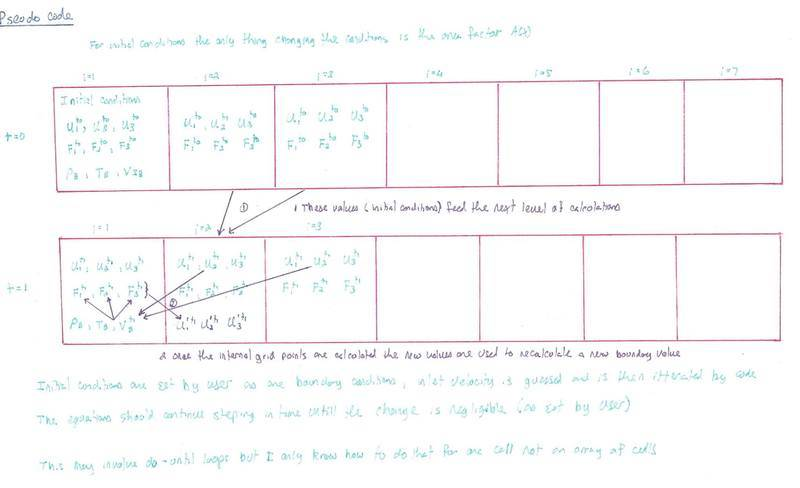# Finite element in MATLAB

• Computational
Does anyone know of a great text that shows how to set up FEA in MATLAB in detail. I have wrote out the sort of pre pseudo code from John Andersons - Computational Fluid Dynamics now I just need to build it out in code. I am old school with the hand sketches, hopefully this does not get me a down vote.#### Attachments

bigfooted
Gold Member
You can also do FEA in matlab in 50 lines:

I thought Anderson also gives some numerical methods in his book?
I would start with a finite difference method if this is your first attempt to write a CFD solver (but matlab is fine, at least you don't have to worry about implementing solvers for sparse matrices).
I don't know a good book on finite element methods, although I have a couple of them on my shelf.

You can also do FEA in matlab in 50 lines:

I thought Anderson also gives some numerical methods in his book?
I would start with a finite difference method if this is your first attempt to write a CFD solver (but matlab is fine, at least you don't have to worry about implementing solvers for sparse matrices).
I don't know a good book on finite element methods, although I have a couple of them on my shelf.

Thank you for the reference. Anderson does not provide raw code, although I did find his email address and asked him (but he has not yet responded). His book does a marvelous job of breaking the PDE's into algebraic problems which is what is required for finite element (PDE -> algebra) and then looped in an array.

Basically I just need to figure out how to define/build out an array and then loop each element of the array in time (allowing each array element to pull values from each other either from adjacent array cells or values from previous time steps in the same array or other arrays.

So the initial array will be pre-populated with values (initial and boundary conditions, the boundary conditions are no longer "neumann" they are simply algebra) and then the calculations will solve and a new array will be created. There will now be 2 arrays with values, the initial conditions (t=0) and the new conditions (t=1), then to get t=2 the calculations will be ran again using the new values from t=1. These new t=2 array values can either replace the initial conditions or just keep creating more arrays (with computing power what it is today and the fact this is a simple pseudo one dimensional problem I don't think there will be issues just to save them but only display the latest values in the plot. I then want to display the values as colors in the shape that I have drawn and have that shape change as I manually change the area factor A(x). This will go on until the new t=x value is negligibly different from t=x-1 (that acceptable difference, steady state, will be set by the user).

I don't think this is super hard to do in matlab but I have never done it so need a little help/reference. I have only done a loop in an "array" with a single box so an array didn't really need to be set up, I simply set up a do until loop to converge on a value.